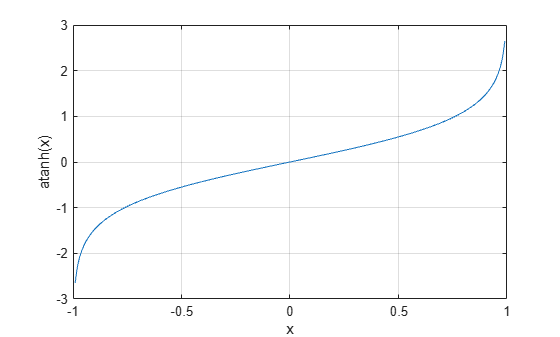# atanh

Inverse hyperbolic tangent

## Syntax

``Y = atanh(X)``

## Description

example

````Y = atanh(X)` returns the inverse hyperbolic tangent of the elements of `X`. The function accepts both real and complex inputs. All angles are in radians.```

## Examples

collapse all

Find the inverse hyperbolic tangent of the elements of vector `X`. The `atanh` function acts on `X` element-wise.

```X = [2 -3 1+2i]; Y = atanh(X)```
```Y = 1×3 complex 0.5493 + 1.5708i -0.3466 - 1.5708i 0.1733 + 1.1781i ```

Plot the inverse hyperbolic tangent function over the interval $-1.

```x = -0.99:0.01:0.99; plot(x,atanh(x)) grid on xlabel('x') ylabel('atanh(x)')```## Input Arguments

collapse all

Hyperbolic tangent of angle, specified as a scalar, vector, matrix, multidimensional array, table, or timetable. The `atanh` operation is element-wise when `X` is nonscalar.

Data Types: `single` | `double` | `table` | `timetable`
Complex Number Support: Yes

collapse all

### Inverse Hyperbolic Tangent

For real values $x$ in the domain $-1, the inverse hyperbolic tangent satisfies

`${\mathrm{tanh}}^{-1}\left(x\right)=\frac{1}{2}\mathrm{log}\left(\frac{1+x}{1-x}\right).$`

For complex numbers $z=x+iy$ as well as real values in the regions $-\text{\hspace{0.17em}}\infty and $1, the call `atanh(z)` returns complex results.

## Version History

Introduced before R2006a

expand all